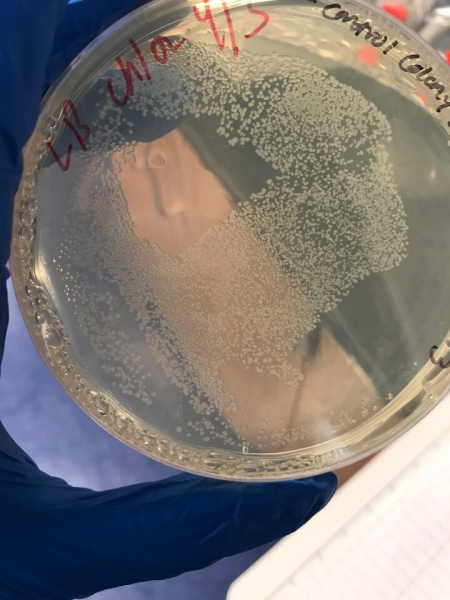# Team:Georgia State/InterLab

At the beginning of this year’s competition an Interlab has been requested to be performed by the iGEM Measurement Committee. Each team had to complete a study and collect data with a given protocol, as well as given supplies such as a LUDOX- CL (colloidal silica suspension), silica beads (microsphere suspension), and the fluorescein protein. The highlight of this Interlab study was to measure the absorbance and fluorescence of a protein called fluorescein as well as using plate readers. The Interlab was divided into three main parts : (1) Calibration protocols, (2) Cell measurements protocol and (3) Calculations of Colony Forming Units (CFU) of E.coli. The goal was to obtain three types of measurements: an OD600 reference point, a particle standard curve and a fluorescein standard curve. Another goal was to be in the exact same conditions as if we were doing our project, to make the calibration valid (same volumes, same plates, temperature, settings, etc..). First, the calibration protocols were performed. The use of microsphere suspension or silica beads was to mimic the cells used in the lab since their size and characteristics were similar. However, since they were given, their amount per volume were known, which helped in the calculation of Abs600. Then the calculation would help determine the concentration of cells, and from the concentration of particles (from the standard curve created), an estimated number of cells was determined. The use of fluorescein was to mimic GFP, however GFP is costly and considerably unstable, so fluorescein was used instead. Fluorescein has similarities with GFP as it has the same emission and excitation properties. The purpose of this measurement was to calibrate the output of fluorescence between the instruments of every teams. With this measurement a standard curve of fluorescein concentration was obtained. Next, the cell measurements were performed. Each team was required to use E.coli K-12 DSH alpha for consistency and reproducibility purposes. The protocol was followed as given by the iGEM Measurement Committee from transformation of the E.coli cells to the pipetting of the colonies in the plate reader. Last, calculating the CFU was the final step of interlab. CFU counts are an indication of the concentration of cells in per mL. The protocol was followed and cells were counted accordingly (See Figure 5).

## Our Data

According to Figure 1 and Figure 2, which gave the particle curve for both trials respectively, the first trial gave the best results. This is due to the trend observed in the curve for Figure 1, it is linear, as opposed to Figure 2. A linear trend indicates that as the number of particles (particle count) increases, the absorbance increases too. This makes sense because the higher the number of particles present, the more they should emit, and the more light should be absorbed. A linear trend is a good indication, and it is an expected result. Figure 2, show a somewhat linear trend, because the curve is not straight enough and it flattens between 0.200 and 0.300 Absorbance at 600 nm. This flat part of the curve, is not a good indication, according to the expected results.

In Figure 3 and 4, the fluorescein standard curve is shown for both trials respectively, as well. Figure 3, shows a linear trend between the fluorescein concentration and the fluorescence. The fluorescence indicates the amount of protein present, through the light emitted by fluorescein . So, a linear trend means that as the fluorescein concentration increases the fluorescence increases as well. This is a good indication, and it is an expected result as well, because the more fluorescein is present, the more light will be emitted, hence the fluorescence would be higher. Figure 4, shows a line that is flat at first, then dramatically increases. This finding is inconsistent with the expected results, since the fluorescein concentration was always increasing. So, the fluorescence should have been increasing as well.Figure 1Figure 2Figure 3Figure 4